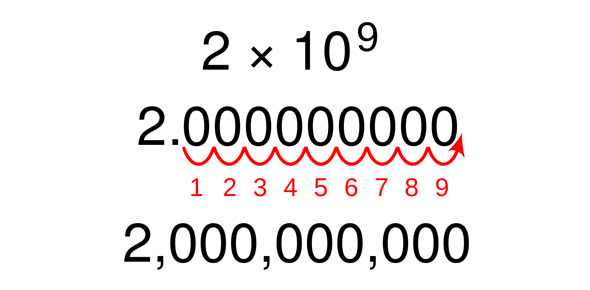# Scientific Notation In Chemistry Quiz Questions

6 Questions | Total Attempts: 449SettingsIf you know scientific notation very good you will certainly get 100.

• 1.
What is the standard form for this? 3,500,000,000
• A.

3.5*10^9

• B.

3*10^9

• C.

3.5*10^8

• 2.
What is the scientific form for this? 9.4*10^8
• A.

940,000,000

• B.

94,000,000,000

• C.

9.4,000,000

• 3.
Is this statment true or false? Is scientific notation good to solve problems with very big numbers and make them very small numbers.
• A.

True

• B.

False

• 4.
Is this number in standard form? 3,400,800,900
• A.

True

• B.

False

• 5.
What is the exponent for this number? 34,000,000,000,000
• A.

10^19

• B.

10^4

• C.

10^13

• 6.
Will the answer have 4 zeros? 5.2*10^4
• A.

True

• B.

False

Related TopicsBack to top